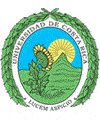``` Universidad de Costa Rica Escuela de Ciencias de la Computación e Informática ```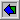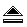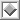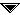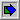# `SelectionSort()`

 ``` var lowkey: keytype; { the currently smallest key found on a pass through A[i], . . . , A[n] } lowindex : integer; { the position of lowkey } begin (1) for i := 1 to n-1 do begin { select the lowest among A[i] ... A[n] and swap it with A[i] } (2) lowindex := i; (3) lowkey := A[i].key; (4) for j := i + 1 to n do { compare each key with current lowkey } (5) if A[j].key < lowkey then begin (6) lowkey := A[j].key; (7) lowindex := j end; (8) swap(A[i], A[lowindex]) end end; ```
Aho, Alfred V.; Hopcroft, John E.; Ullman, Jefrrey D.
"Data Structures and Algorithms"; Addisson Wesley Publishing Co.; 1984.